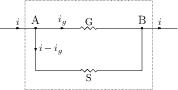# Moving Coil Galvanometer, Voltmeter, Ammeter, and their Conversions

## Problems from IIT JEE

Problem (IIT JEE 2008): Statement 1: The sensitivity of a moving coil galvanometer is increased by placing a suitable magnetic material as core inside the coil.

Statement: Soft iron has a high magnetic permeability and cannot be easily magnetized or demagnetized.

1. Statement 1 is true, statement 2 is true; statement 2 is a correct explanation for statement 1.
2. Statement 1 is true, statement 2 is true; statement 2 is not a correct explanation for statement 1.
3. Statement 1 is true, statement 2 is false.
4. Statement 1 is false, statement 2 is true.

Solution: The sensitivity of a moving-coil galvanometer is defined as angular deflection $\theta$ per unit current $i$. It is given as $\theta/i=nAB/k$, where $n$ is number of turns, $A$ is coil area, $B$ is magnetic field, and $k$ is torsional constant of suspension wire. To increase sensitivity, $B$ is increased by placing a soft iron core inside the coil. The soft iron has a high magnetic permeability and can be easily magnetized or demagnetized.

Problem (IIT JEE 2005): A moving coil galvanometer of resistance ${100}\;{\Omega}$ is used as an ammeter using a resistance ${0.1}\;{\Omega}$. The maximum deflection current in the galvanometer is ${100}\;{\mu A}$. Find the current in the circuit, so that the ammeter shows maximum deflection,

1. 100.1 mA
2. 1000.1 mA
3. 10.01 mA
4. 1.01 mA

Solution:A galvanometer of resistance $G$ is converted to an ammeter by connecting a small shunt resistance $S$ in parallel (see figure). Kirchhoff's loop law gives, \begin{align} &i_gG-(i-i_g)S=0,\quad \implies \quad i=i_g(G+S)/S. \nonumber \end{align} The maximum deflection current of galvanometer sets upper limit on the current measured by this ammeter. Substitute the values to get, \begin{alignat}{2} &i=i_g({G+S})/{S}=(100\times{10}^{-6})\left(({100+0.1})/{0.1})\right)={100.1}\;\mathrm{mA}. \nonumber \end{alignat}